Myths and Misconceptions

So it has been a long time since I have written any Guide so I thought to write a guide today about some commons myths and misconceptions which are present here.
Let’s get started with a basic one.

### Logic/Math/Text Equal To blocks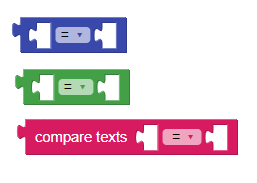We have seen a lot of posts in community that which one should use and which not.
But first a brief introduction.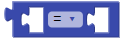is a condition block present in the Math category which is mainly used to compare two numbers.is a condition block present in the Logic category which is mainly used to compare two objects.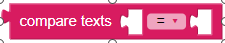is a condition block present in the Text category which is mainly used to compare two texts.

So what is misconception here???To understand it, look at below Do it results.

You can easily notice the results are different for Text Equal To block and the results for Math and Logic Equal To blocks are same.
We can conclude from the above test that:

• Text Equal To block should be used only when both inputs have the same alphabet and in the same order
• Logic and Math Equal To blocks can be used for any type of comparison.

### Open Screen Methods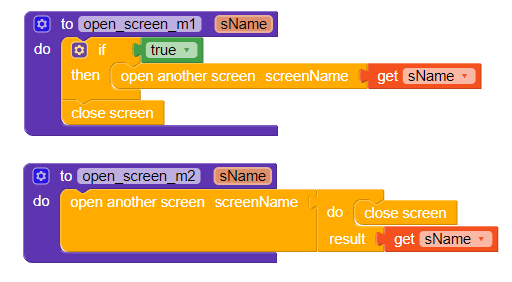Now you will ask what the myth is here.
A common myth about these methods is that both methods are same and can be interchanged.
But the fact is that both are different.What? Yes, you read it right.
Both are very different.
And here is the reason why:

• The first method will open the screen first and then close the active screen.
In some cases, it has been seen that it closes the newly opened screen instead of the older one because the `Close Screen` block/method is executed in new Screen.
• The second method will close the active screen first and then open the new screen.
However it is opposite in the case of Java: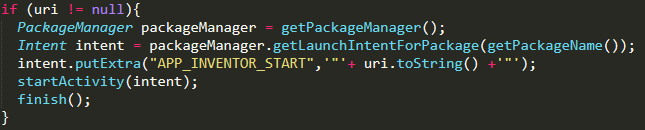You can ask what if the active screen is Screen1?It should close the application in that case.
The answer is that nothing will happen because to close an application `System.exit(0)` should be called which `CloseScreen` method does not call.

Hope it helps!

Subscribe
Notify of